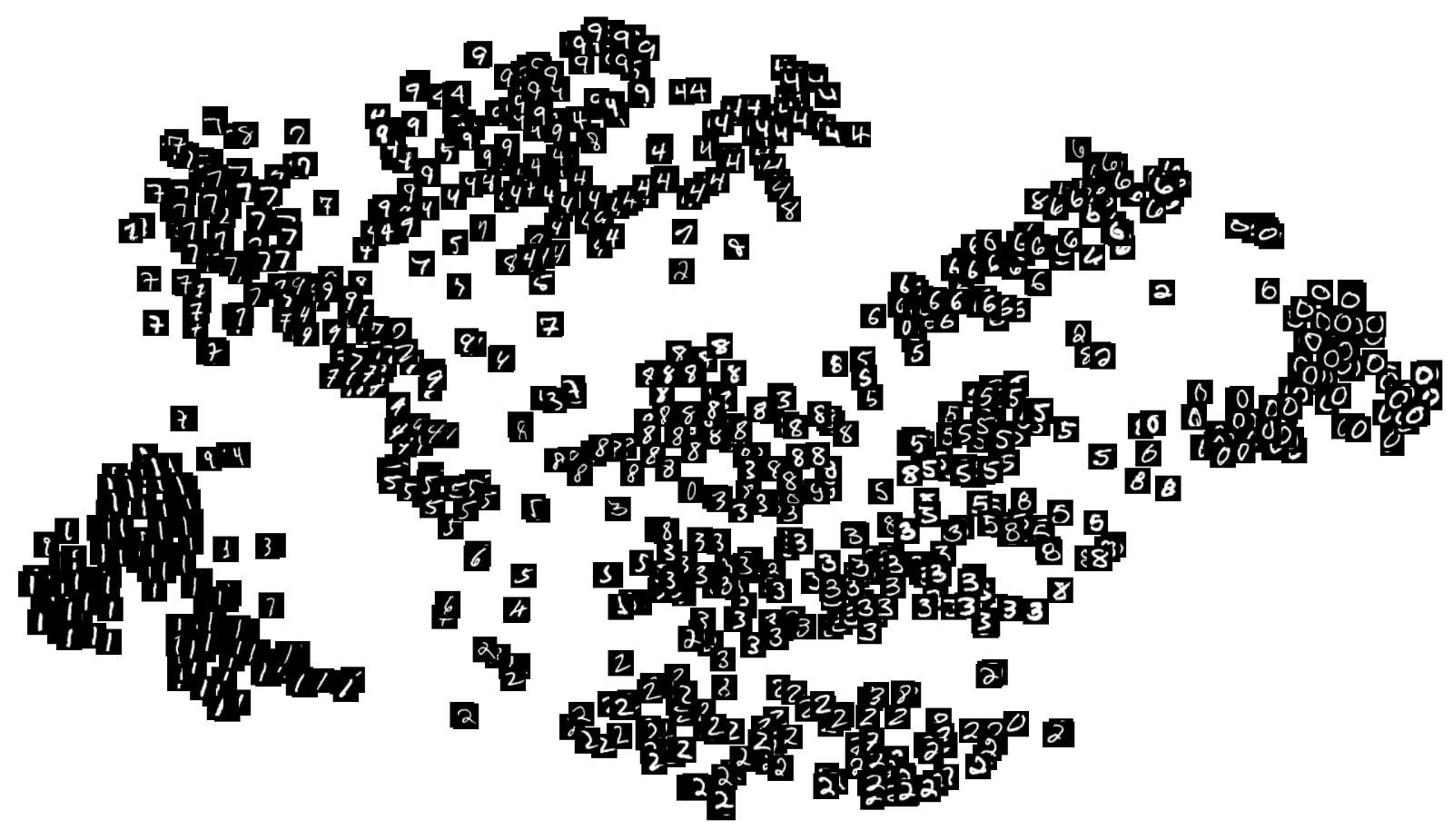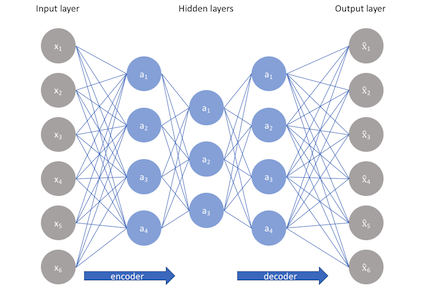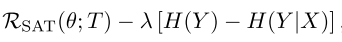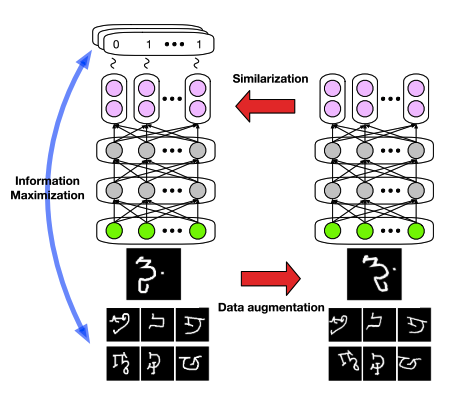# An Overview of Deep Learning Based Clustering TechniquesThis post gives an overview of various deep learning based clustering techniques. I will be explaining the latest advances in unsupervised clustering which achieve the state-of-the-art performance by leveraging deep learning.

Unsupervised learning is an active field of research and has always been a challenge in deep learning. Finding out meaningful patterns from large datasets without the presence of labels is extremely helpful for many applications. Advances in unsupervised learning are very crucial for artificial general intelligence. Performing unsupervised clustering is equivalent to building a classifier without using labeled samples.

In the past 3-4 years, several papers have improved unsupervised clustering performance by leveraging deep learning. Several models achieve more than 96% accuracy on MNIST dataset without using a single labeled datapoint. However, we are still very far away from getting good accuracy for harder datasets such as CIFAR-10 and ImageNet.

In this post, I will be covering all the latest clustering techniques which leverage deep learning. The goal of most of these techniques is to clusters the data-points such that the data-points of the same ground truth class are assigned the same cluster. The deep learning based clustering techniques are different from traditional clustering techniques as they cluster the data-points by finding complex patterns rather than using simple pre-defined metrics like intra-cluster euclidean distance.

## Clustering with unsupervised representation learning

One method to do deep learning based clustering is to learn good feature representations and then run any classical clustering algorithm on the learned representations. There are several deep unsupervised learning methods available which can map data-points to meaningful low dimensional representation vectors. The representation vector contains all the important information of the given data-point, hence clustering on the representation vectors yield better results.

One popular method to learn meaningful representations is deep auto-encoders. Here the input is fed into a multilayer encoder which has a low dimensional output. That output is fed to a decoder which produces an output of the same size as input. The training objective of the model is to reconstruct the given input. In order to do that successfully, the learned representations from the encoder contain all the useful information compressed in a low dimensional vector.Running K-means on representation vectors learned by deep autoencoders tend to give better results compared to running K-means directly on the input vectors. For example in MNIST, clustering accuracy of K Means is 53.2% while running K-means on the learned representations from auto-encoders yield an accuracy of 78.9%.

Other techniques to learn meaningful representations include :

## Clustering via information maximization

Regularized Information Maximization is an information theoretic approach to perform clustering which takes care of class separation, class balance, and classifier complexity. The method uses a differentiable loss function which can be used to train multi-logit regression models via backpropagation. The training objective is to maximize the mutual information between the input x and the model output y while imposing some regularisation penalty on the model parameters.

Mutual information can be represented as the difference between marginal entropy and conditional entropy. Hence the training objective to minimize is :Here it is maximizing the marginal entropy H(Y) and minimizing the conditional entropy H( Y|X ).

By maximizing H(Y), the cluster assignments are diverse, hence the model cannot degenerate by assigning a single cluster to all the input data points. In fact, it will try to make the distribution of clusters as uniform as possible because entropy will be maximum when the probability of each cluster is the same.

The neural network model with the softmax activation estimates the conditional probability p( y|x ). By minimizing H( Y|X ), it ensures that the cluster assignment of any data point is with high confidence. If H( Y|X ) is not minimized and only H(Y) is maximized, the model can degenerate by assigning an equal conditional probability to each cluster given any input.

While implementing in order to compute H(Y), p(y) is computed by marginalizing p( y|x ) over a mini-batch. For a given x, p( y|x ) is the output of the network after the softmax activation.

## Information maximization with self augmented training.

The method described above assigns clusters while trying to balance the number of data points in the clusters. The only thing which tries to ensure the cluster assignments to be meaningful is the regularization penalty on the model parameters. A better way to ensure the cluster assignments to be meaningful is to have a way such that similar data-points go to the same clusters.

Information maximization with self augmented training ( IMSAT) is an approach which uses data augmentation to generate similar data-points. Given a data point x, an augmented training example T(x) is generated where T : XX denote a pre-defined data augmentation function. The cross entropy between p( y|x ) and p( y|T(x) ) is minimized.The authors of IMSAT propose two ways to augment the data and train the model : 1) Random Perturbation Training 2) Virtual Adversarial Training

Random Perturbation Training ( RPT ) : Here a random perturbation r from a pre-defined noise distribution is added to the input. Hence the, augmentation function will be T(x) = x + r . The random perturbation r is chosen randomly from hyper-sphere. As you can see, this is a very naive way to do augmentation.

Virtual Adversarial Training ( VAT ) : Here, rather than randomly choosing the perturbation randomly, the perturbation is chosen such that the model fails to assign them to the same cluster. A limit is imposed on the perturbation r so that input is not changed a lot.

This training is somewhat similar to how GANs are trained. Rather than having a generator fooling the discriminator, we generate a perturbation such the model is fooled in assigning the pair different clusters. Simultaneously it makes the model better and it does not make the same mistake in the future. The paper reports significant improvement in VAT over RPT for some datasets.

Deep adaptive clustering ( DAC ) uses a pairwise binary classification framework. Given two input data-points, model outputs whether the inputs belong to the same cluster or not. Basically, there is a network with a softmax activation which takes an input data-point and produces a vector with probabilities of the input belong to the given set of clusters. Given two input data-points, the dot product of the model outputs of both the inputs is taken. When the cluster assignments of the two inputs are different, the dot product will be zero and for the same cluster assignments, the dot product will be one. As dot product is a differentiable operation, we can train it with backpropagation with pairwise training labels.As the ground truth data is not available, the features of the same network are used to create binary labels for the pairwise training. Cosine distance between the features of the two data-points is used. Given an input pair, if the cosine distance is greater than the upper threshold, then the input pair is considered a positive pair ( meaning both should be in the same cluster). Similarly, if the cosine distance is lesser than the lower threshold then the input pair is considered a negative pair ( meaning both should be in different clusters ). If the distance lies between the lower threshold and the upper threshold, the pair is ignored. After getting the positive and the negative pairs, the pairwise loss is minimized.

As the pairwise loss is minimized, it becomes better in classifying pair of data-points and the features of the network become more meaningful. With features becoming more meaningful, the binary labels obtained via cosine distance of the features become more accurate.

You may think this as a chicken and egg problem and the question is how to get a good start. The solution is having a good random initialization distribution. With standard initialization techniques, even with random model weights output is related to the inputs ( behaving like extreme learning machine ). Hence cosine distance of the features is somewhat meaningful in the beginning. In the beginning, the upper threshold is set to a large value as the cosine distance measure is not very accurate. Over iterations, the upper threshold is decreased.

## Conclusion

I hope this post was able to give you an insight into various deep learning based clustering techniques. The deep learning based methods have outperformed traditional clustering techniques in many benchmarks. Most of the methods discussed are promising and there is huge potential for improvement in several datasets. If you have any questions or want to suggest any changes feel free to contact me or write a comment below.